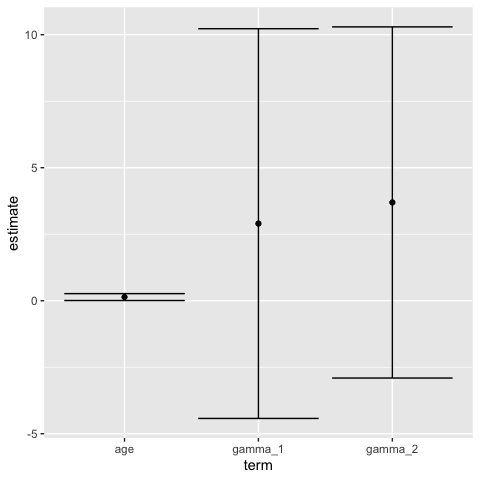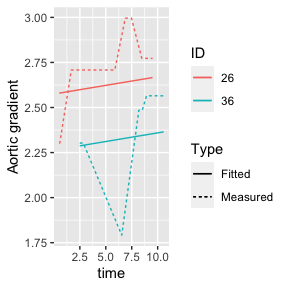# Introduction

Tidiers for objects of class mjoint have been included in latest release of joineRML package (0.4.5). The purpose of these tidiers are described in the introductory vignette to broom:

The broom package takes the messy output of built-in functions in R, such as lm, nls, or t.test, and turns them into tidy data frames.

There are three distinct tidiers included with broom:

• tidy: constructs a data frame that summarises the model estimates;

• augment: add columns to the original data that was modeled;

• glance: construct a concise one-row summary of the model.

These methods are specifically useful when plotting results of a joint model or when comparing several models (e.g. in terms of fit).

# Example

We use the sample example from the introductory vignette to joineRML using the heart valve data.

vignette("joineRML", package = "joineRML")
help("heart.valve", package = "joineRML")

We analyse only the records with case-complete data for heart valve gradient (grad) and left ventricular mass index (lvmi):

data(heart.valve)
hvd <- heart.valve[!is.na(heart.valve$grad) & !is.na(heart.valve$lvmi), ]

Further to that, we only select the first 50 individuals to speed up these examples:

hvd <- hvd[hvd$num <= 50, ] set.seed(12345) fit <- mjoint( formLongFixed = list( "grad" = log.grad ~ time + sex + hs, "lvmi" = log.lvmi ~ time + sex ), formLongRandom = list( "grad" = ~ 1 | num, "lvmi" = ~ time | num ), formSurv = Surv(fuyrs, status) ~ age, data = list(hvd, hvd), timeVar = "time" ) ## Running multivariate LMM EM algorithm to establish initial parameters... ## Finished multivariate LMM EM algorithm... ## EM algorithm has converged! ## Calculating post model fit statistics... ## tidy method The tidy method returns a tidy dataset with model estimates. tidy(fit) ## # A tibble: 3 × 5 ## term estimate std.error statistic p.value ## <chr> <dbl> <dbl> <dbl> <dbl> ## 1 age 0.137 0.0661 2.07 0.0382 ## 2 gamma_1 2.90 3.74 0.776 0.438 ## 3 gamma_2 3.69 3.37 1.10 0.273 By default the tidy method returns the estimated coefficients for the survival component of the joint model; however, it is possible to extract each component by setting the component argument: tidy(fit, component = "longitudinal") ## # A tibble: 7 × 5 ## term estimate std.error statistic p.value ## <chr> <dbl> <dbl> <dbl> <dbl> ## 1 (Intercept)_1 2.52 0.163 15.4 1.07e-53 ## 2 time_1 0.00959 0.0310 0.309 7.57e- 1 ## 3 sex_1 0.0336 0.230 0.146 8.84e- 1 ## 4 hsStentless valve_1 0.0823 0.198 0.416 6.77e- 1 ## 5 (Intercept)_2 4.99 0.0829 60.2 0 ## 6 time_2 0.0260 0.0129 2.02 4.36e- 2 ## 7 sex_2 -0.197 0.209 -0.941 3.47e- 1 It is also possible to require confidence intervals to be calculated by setting conf.int = TRUE, and modify the confidence level by setting the conf.level argument: tidy(fit, ci = TRUE) ## # A tibble: 3 × 5 ## term estimate std.error statistic p.value ## <chr> <dbl> <dbl> <dbl> <dbl> ## 1 age 0.137 0.0661 2.07 0.0382 ## 2 gamma_1 2.90 3.74 0.776 0.438 ## 3 gamma_2 3.69 3.37 1.10 0.273 tidy(fit, ci = TRUE, conf.level = 0.99) ## # A tibble: 3 × 5 ## term estimate std.error statistic p.value ## <chr> <dbl> <dbl> <dbl> <dbl> ## 1 age 0.137 0.0661 2.07 0.0382 ## 2 gamma_1 2.90 3.74 0.776 0.438 ## 3 gamma_2 3.69 3.37 1.10 0.273 The standard errors reported by default are based on the empirical information matrix, as in mjoint. It is of course possible to use bootstrapped standard errors as follows: bSE <- bootSE(fit, nboot = 100, safe.boot = TRUE, progress = FALSE) tidy(fit, boot_se = bSE, conf.int = TRUE) The results of this example are not included as it would take too long to run for CRAN. The tidy method is useful for custom plotting (e.g. forest plots) of results from joineRML models, all in a tidy framework: library(ggplot2) out <- tidy(fit, conf.int = TRUE) ggplot(out, aes(x = term, y = estimate, ymin = conf.low, ymax = conf.high)) + geom_point() + geom_errorbar()## augment method The augment method returns a dataset with added predictions from the joint model. In particular, population-level and individual-level fitted values and residuals are added to the data frame returned by the method: preds <- augment(fit) head(preds[, c("num", "log.grad", ".fitted_grad_0", ".fitted_grad_1", ".resid_grad_0", ".resid_grad_1")]) ## # A tibble: 6 × 6 ## num log.grad .fitted_grad_0 .fitted_grad_1 .resid_grad_0 .resid_grad_1 ## <fct> <dbl> <dbl> <dbl> <dbl> <dbl> ## 1 1 2.30 2.60 2.42 -0.301 -0.114 ## 2 1 2.30 2.64 2.45 -0.337 -0.149 ## 3 1 2.30 2.65 2.46 -0.346 -0.158 ## 4 2 2.64 2.58 2.45 0.0563 0.188 ## 5 2 2.20 2.59 2.46 -0.394 -0.263 ## 6 2 2.48 2.60 2.47 -0.116 0.0152 head(preds[, c("num", "log.lvmi", ".fitted_lvmi_0", ".fitted_lvmi_1", ".resid_lvmi_0", ".resid_lvmi_1")]) ## # A tibble: 6 × 6 ## num log.lvmi .fitted_lvmi_0 .fitted_lvmi_1 .resid_lvmi_0 .resid_lvmi_1 ## <fct> <dbl> <dbl> <dbl> <dbl> <dbl> ## 1 1 4.78 4.99 4.75 -0.213 0.0298 ## 2 1 4.78 5.09 4.87 -0.308 -0.0869 ## 3 1 4.92 5.11 4.90 -0.189 0.0265 ## 4 2 4.74 5.16 4.83 -0.413 -0.0827 ## 5 2 4.70 5.18 4.86 -0.483 -0.157 ## 6 2 5.06 5.21 4.89 -0.149 0.174 We can plot the resulting predictions for four distinct individuals: out <- preds[preds$num %in% c(26, 36, 227, 244), ]
ggplot(out, aes(x = time, colour = num)) +
geom_line(aes(y = log.grad, linetype = "Measured")) +
geom_line(aes(y = .fitted_grad_1, linetype = "Fitted")) +
labs(linetype = "Type", colour = "ID", y = "Aortic gradient")## glance method

The glance method allows extracting summary statistics from the joint model:

glance(fit)
## # A tibble: 1 × 5
##   sigma2_1 sigma2_2   AIC   BIC logLik
##      <dbl>    <dbl> <dbl> <dbl>  <dbl>
## 1    0.517    0.158  342.  374.  -153.

This allows comparing competing models easily. Say for instance that we fit a second model with only random intercepts:

set.seed(67890)
fit2 <- mjoint(
formLongFixed = list(
"lvmi" = log.lvmi ~ time + sex
),
formLongRandom = list(
"grad" = ~ 1 | num,
"lvmi" = ~ 1 | num
),
formSurv = Surv(fuyrs, status) ~ age,
data = list(hvd, hvd),
timeVar = "time"
)
## Running multivariate LMM EM algorithm to establish initial parameters...
## Finished multivariate LMM EM algorithm...
## EM algorithm has converged!
## Calculating post model fit statistics...

We can go ahead and compare the models in terms of AIC and BIC:

glance(fit)
## # A tibble: 1 × 5
##   sigma2_1 sigma2_2   AIC   BIC logLik
##      <dbl>    <dbl> <dbl> <dbl>  <dbl>
## 1    0.517    0.158  342.  374.  -153.
glance(fit2)
## # A tibble: 1 × 5
##   sigma2_1 sigma2_2   AIC   BIC logLik
##      <dbl>    <dbl> <dbl> <dbl>  <dbl>
## 1    0.516    0.173  342.  369.  -156.

Several examples of how to use broom including more details are available on its introductory vignette:
vignette(topic = "broom", package = "broom")